Circle, Disk

The basic use of Circle is demonstrated with the next cell.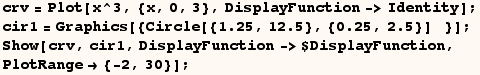The next cell demonstrates a circle with radi specified using scaled coordinates.  In this case the radius is 1/4 the width of the graphic in the horizontal direction and 1/4 of the height of the graphic in the vertical direction.  Hence the diameter is 1/2 the size of the graphic in each direction.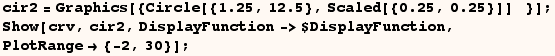The next cell makes a circle with radi given as a combination of scaled coordinates and absolute coordinates.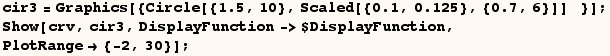The documentation for Circle indicates Offset[{ax,ay}] can be used to specify radii in printer points.  This is demonstrated below where the circle is round with a radius of 15 printer points regardless of the AspectRatio or ImageSize.  You can even resize the graphic, and the size of the circle will not change!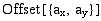can also be used to specify radius of a Disk with similar results.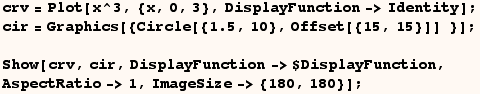Created by Mathematica  (May 16, 2004)

Back to Ted’s Tricks index page